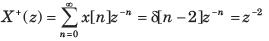Courses

# Test: Z-Transform Part - 1

## 10 Questions MCQ Test Signal and System | Test: Z-Transform Part - 1

Description
This mock test of Test: Z-Transform Part - 1 for Electrical Engineering (EE) helps you for every Electrical Engineering (EE) entrance exam. This contains 10 Multiple Choice Questions for Electrical Engineering (EE) Test: Z-Transform Part - 1 (mcq) to study with solutions a complete question bank. The solved questions answers in this Test: Z-Transform Part - 1 quiz give you a good mix of easy questions and tough questions. Electrical Engineering (EE) students definitely take this Test: Z-Transform Part - 1 exercise for a better result in the exam. You can find other Test: Z-Transform Part - 1 extra questions, long questions & short questions for Electrical Engineering (EE) on EduRev as well by searching above.
QUESTION: 1

###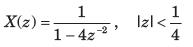Solution: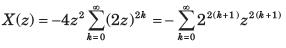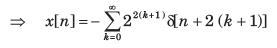QUESTION: 2

### X (z) = ln (1 + z-1 ), |z|>0

Solution: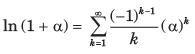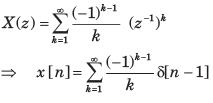QUESTION: 3

### If z-transform is given by X (z) = cos ( z -3), |z|> 0, The value of x is

Solution: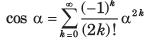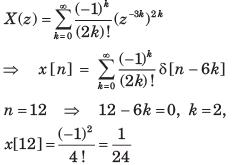QUESTION: 4

X (z) of a system is specified by a pole zero pattern in fig. P.5.4.36.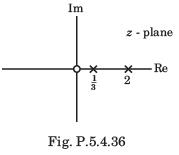Consider three different solution of x[n]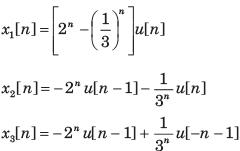Correct solution is

Solution:

All gives the same z transform with different ROC. So all are the solution.

QUESTION: 5

Consider three different signal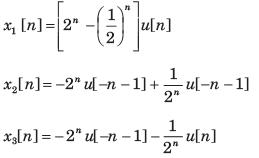Fig. P.5.4.37 shows the three different region. Choose the correct option for the ROC of signal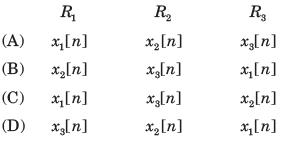Solution:

x1[n] is right-sided signal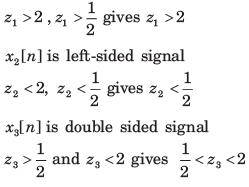QUESTION: 6

Given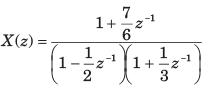For three different ROC consider there different solution of signal x[n] :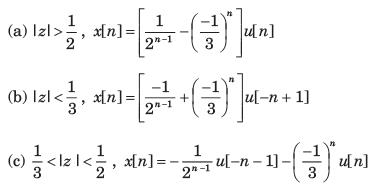Correct solutions are

Solution: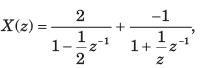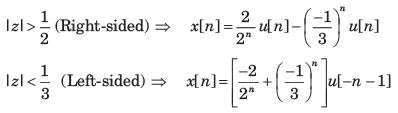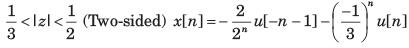So (b) is wrong.

QUESTION: 7

X (z) has poles at z = 1/2 and z = -1. If x  = 1 x [ -1] = 1, and the ROC includes the point z = 3/4. The time signal x[n] is

Solution:

Since the ROC includes the Z = 3/4, ROC is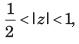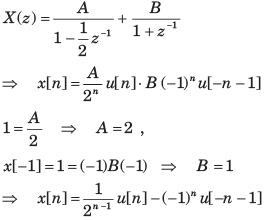QUESTION: 8

x[n] is right-sided, X (z) has a signal pole, and x = 2, x = 1/2. x[n] is

Solution:

x[n]= Cpnu[n],x[0=2=C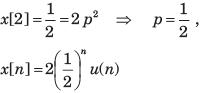QUESTION: 9

The z-transform function of a stable system is given as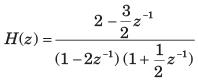The impuse response h[n] is

Solution: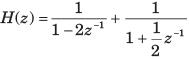h[n] is stable, so ROC includes |z|= 1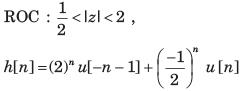QUESTION: 10

Let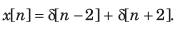The unilateral z-transform is

Solution: